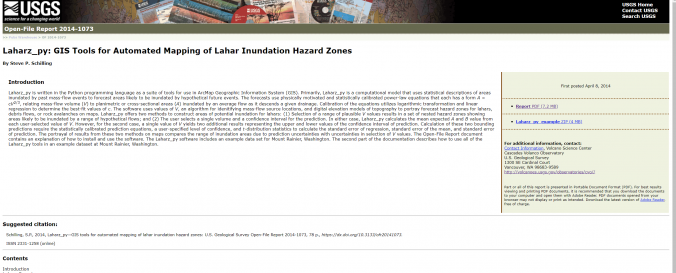# GIS Tools for Automated Mapping of Lahar Inundation Hazard ZonesDescription: Laharz_py is written in the Python programming language as a suite of tools for use in ArcMap Geographic Information System (GIS). Primarily, Laharz_py is a computational model that uses statistical descriptions of areas inundated by past mass-flow events to forecast areas likely to be inundated by hypothetical future events. The forecasts use physically motivated and statistically calibrated power-law equations that each has a form A = cV2/3, relating mass-flow volume (V) to planimetric or cross-sectional areas (A) inundated by an average flow as it descends a given drainage. Calibration of the equations utilizes logarithmic transformation and linear regression to determine the best-fit values of c. The software uses values of V, an algorithm for idenitifying mass-flow source locations, and digital elevation models of topography to portray forecast hazard zones for lahars, debris flows, or rock avalanches on maps. Laharz_py offers two methods to construct areas of potential inundation for lahars: (1) Selection of a range of plausible V values results in a set of nested hazard zones showing areas likely to be inundated by a range of hypothetical flows; and (2) The user selects a single volume and a confidence interval for the prediction. In either case, Laharz_py calculates the mean expected A and B value from each user-selected value of V. However, for the second case, a single value of V yields two additional results representing the upper and lower values of the confidence interval of prediction. Calculation of these two bounding predictions require the statistically calibrated prediction equations, a user-specified level of confidence, and t-distribution statistics to calculate the standard error of regression, standard error of the mean, and standard error of prediction. The portrayal of results from these two methods on maps compares the range of inundation areas due to prediction uncertainties with uncertainties in selection of V values. The Open-File Report document contains an explanation of how to install and use the software. The Laharz_py software includes an example data set for Mount Rainier, Washington. The second part of the documentation describes how to use all of the Laharz_py tools in an example dataset at Mount Rainier, Washington. Software type: Tool/Converter Website: https://pubs.usgs.gov/of/2014/1073/ Accessibility: Install only Requirements: Requires ArcGIS with the spatial analyst extension Computer System: Windows Scope: Modelling Vector/Raster: Vector, Raster Graphical user interface: Yes Costs: Free Tutorials: Lahar Modeling Using Laharz Toolbox ArcGIS